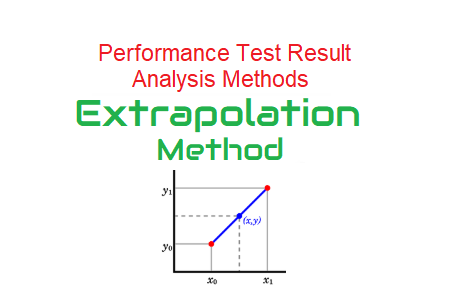# Extrapolation MethodAs per definition, Extrapolate refers to extend the application of (a method or conclusion) to an unknown situation by assuming that existing trends will continue or similar methods will be applicable. In Performance Testing, Extrapolation is required when an application is tested on a scaled-down environment with lesser number of users. As per the capacity of the scaled-down environment server, an appropriate load is applied and then results are extended as per production servers. This method is called as ‘Extrapolation Method’.

Extrapolation Method predicts the production server capacity based on test server result. Many clients do not afford the cost of 100% scaled performance test environment. Hence either they ask to execute the test in 50% scaled-down environment or using one instance of the server. In such a case, a performance tester needs to execute the test in the provided environment which has scaled-down resources. One important point to be noted here all the resources of the servers should have the same scaled-down ratio. Also, the same technique and calculation should be used for extrapolating the results. An uneven ratio or different techniques may lead to the wrong extrapolation result.

### How to apply Extrapolation Method?

Some important points before executing the test and applying the Extrapolation Method:

• Complete the basic tuning of the application.
• No software bottlenecks such as locks, sockets, connection pools or thread pools.
• No performance issue in the network or related to network bandwidth
• The configurations of Production and test environment should be in a ratio
Example: Let’s say production has 4 Web servers, 2 app servers and 2 DB servers. Then the test environment should have 2 Web servers, 1 app server and 1 DB server
Or
Take a single “leg” deployment of the application infrastructure i.e. if there are 3 web servers, 3 app servers and a DB cluster then configure 1 web, 1 app and the DB cluster. Arguably, you could do this with any subset of the full deployment model. But it is better to do this using the smallest practical subset.
• JVM heap memory should be in ratio.
• Log setting of test and prod server should be the same
• Verify the connection limits of all servers in the test environment which should have the same scaled-down ratio as the production environment.

Generally, Linear regression method is useful in many practical applications for extending an approximately linear function to points close to existing data points. However, the technique may cause a larger error for predicting results farther off from the existing data points. Its advantages are computational simplicity and ease of application. Linear regression assumes that the past trend continues in the future and the past and present data series embed the entire information regarding the data trend. However, this does not take into account any external conditions or constraints. If it fails due to certain conditions the past trend of data series does not continue.

### Linear Extrapolation Method Approach:

The linear extrapolation method requires at least two sets of the result. The more sets of result you have, the more accuracy in the prediction result. Firstly, you have to prepare a step-up test scenario and execute the test. There are two ways, either you can run multiple tests by gradually increasing the load in each test or you can set multiple load enhancement steps in the same test. Now, execute the test(s) whichever meet(s) your requirement and get the result.

To understand the extrapolation method more practically, let’s take one example. I executed a test which had gradually increase in the load. Before a system encounters any bottleneck, the throughput increased linearly with the number of concurrent users. In such a scenario, each user was going to receive additional pages from the server thus leading to an increase in the total throughput at a constant rate (linear increase). This indicates that linear extrapolation is an obvious choice for predicting the throughput of a system until the system encounters a bottleneck. I noted down the throughput at two levels of the load and want to predict what would be the throughput at 200 user load?

Hence at 200 user load, the predicted throughput would be 199K.

### Linear Extrapolation Calculator:

where,
x1 and x2 are random values on the x-axis and y1 and y2 are respective values on the y-axis. X represents the targetted value and Y represents the extrapolated value.

### Tips:

• You can use Linear Extrapolation for Throughput, Hits per seconds, TPS, Java Heap Size etc.
• For Response Time, Latency, CPU Utilization and Memory Utilization, it is better to use S-curves or Mixed mode technique.

### Conclusion:

Stating blindly that if the server handles 500 users load with half of the production configuration then the production server can handle 1000 users load; is incorrect. Such a report has a risk of failure of the whole production system even at normal user load. So, when you execute the test on a scaled-down environment then get the result, apply the appropriate extrapolation method and predict the correct metric.

You may be interested:

### 2 Responses

1.Kumar says:

Can we get the formula also for this calculation part from the calculator

•PerfMatrix says:

The formula is based on simple ratio logic.